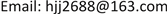1徐州阿马凯液压技术有限公司，江苏 徐州

2徐州重型机械有限公司，江苏 徐州1. 前言

2. 阀前补偿的负载敏感系统

Q 2 = C d A 2 2 ρ Δ P 2 (2)

Δ P 1 = P a − P 1 (3)

Δ P 2 = P b − P 2 (4)

P a = P 1 + Δ X 1 (5)

P b = P 2 + Δ X 2 (6)

Δ P 1 = Δ X 1 (7)

Δ P 2 = Δ X 2 (8)

2.1. 流量非饱和状态

2.2. 流量饱和状态

3. 阀后补偿的负载敏感系统

Q 11 = C d A 1 2 ρ Δ P 1 (9)

Q 22 = C d A 2 2 ρ Δ P 2 (10)

Δ P 1 = P s − P a 1 (11)

Δ P 2 = P s − P b 1 (12)

P a 1 = P m + P k (13)

P b 1 = P m + P k (14)

P k ——压力补偿阀弹簧腔由弹簧所产生的压力(阀后补偿多路阀中压力补偿阀弹簧一样)。

3.1. 流量非饱和状态

3.2. 流量饱和状态

4. 结论

1) 在流量非饱和状态下，无论单动作或复合动作，阀前负载敏感与阀后负载敏感技术均能满足其性能要求，从价格、可靠性、节能等方面考虑，建议采用阀前负载敏感技术。

2) 在流量饱和状态下，虽然有复合动作的需求，但对复合动作的协调性方面没有特殊要求或无要求，阀前负载敏感和阀后均能满足系统要求，建议采用阀前负载敏感技术。

3) 在流量饱和状态下，对复合动作的协调性有严格要求，在此工况下阀前敏感技术失效，只能采用阀后敏感技术。